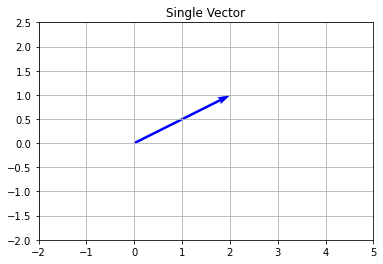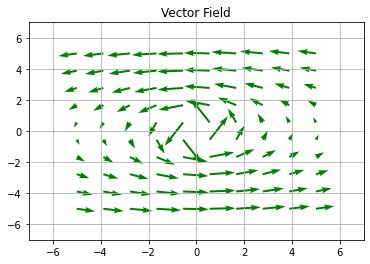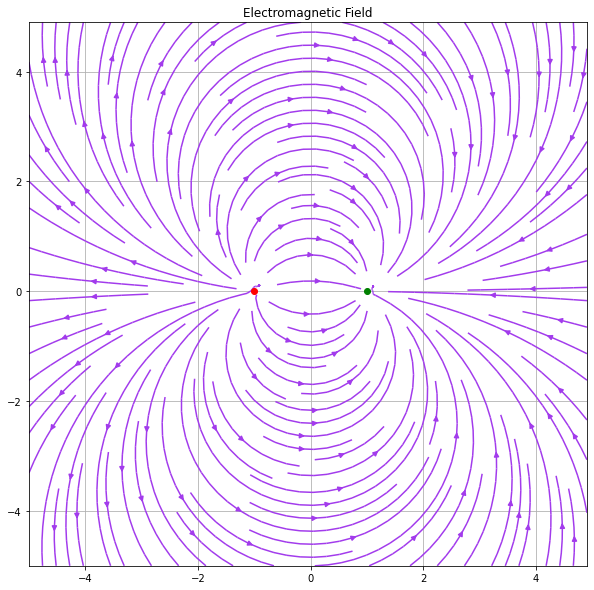# How to plot a simple vector field in Matplotlib ?

The quantity incorporating both magnitude and direction is known as Vectors. In simple words, we can say, Vector Field is an engagement or collaboration of such vectors in a subset of space. Vector fields are the key aspects of understanding our real-life surrounding.

For more intuition, you can think of a vector field as representing a multivariable function whose input and output spaces each have the same dimension. The length of arrows drawn in a vector field is usually not to scale, but the ratio of the length of one vector to another should be accurate.

In this article, we are going to discuss how to plot a vector field in python. In order to perform this task we are going to use the quiver() method and the streamplot() method in matplotlib module.

Syntax:

To plot a vector field using the quiver() method:

matplotlib.pyplot.quiver(X, Y, U, V, **kw)

Where X, Y define the Vector location and U, V are directional arrows with respect of the Vector location.

To plot a vector field using the streamplot() method:

matplotlib.pyplot.streamplot(X, Y, U, V, density=1, linewidth=None, color=None, **kw)

Where X, Y are evenly spaced grid[1D array] and U and V represent the stream velocity of each point present on the grid. Density is the no. of vector per area of the plot. Line width represents the thickness of streamlines.

Below are some examples which depict how to plot vector fields using matplotlib module:

Example 1: Plotting a single vector using quiver() method in matplotlib module.

## Python3

 `# Import libraries ` `import` `numpy as np ` `import` `matplotlib.pyplot as plt ` ` `  `# Vector origin location ` `X ``=` `[``0``] ` `Y ``=` `[``0``] ` ` `  `# Directional vectors ` `U ``=` `[``2``]   ` `V ``=` `[``1``]   ` ` `  `# Creating plot ` `plt.quiver(X, Y, U, V, color``=``'b'``, units``=``'xy'``, scale``=``1``) ` `plt.title(``'Single Vector'``) ` ` `  `# x-lim and y-lim ` `plt.xlim(``-``2``, ``5``) ` `plt.ylim(``-``2``, ``2.5``) ` ` `  `# Show plot with grid ` `plt.grid() ` `plt.show() `

Output:Example 2: Generating multiple vectors using quiver() method.

## Python3

 `# Import required modules ` `import` `numpy as np ` `import` `matplotlib.pyplot as plt ` ` `  `# Meshgrid ` `x, y ``=` `np.meshgrid(np.linspace(``-``5``, ``5``, ``10``),  ` `                   ``np.linspace(``-``5``, ``5``, ``10``)) ` ` `  `# Directional vectors ` `u ``=` `-``y``/``np.sqrt(x``*``*``2` `+` `y``*``*``2``) ` `v ``=` `x``/``(x``*``*``2` `+` `y``*``*``2``) ` ` `  `# Plotting Vector Field with QUIVER ` `plt.quiver(x, y, u, v, color``=``'g'``) ` `plt.title(``'Vector Field'``) ` ` `  `# Setting x, y boundary limits ` `plt.xlim(``-``7``, ``7``) ` `plt.ylim(``-``7``, ``7``) ` ` `  `# Show plot with grid ` `plt.grid() ` `plt.show() `

Output:Example 3: Plotting multiple vectors using streamplot() method in matplotlib module.

## Python3

 `# Import required modules ` `import` `numpy as np ` `import` `matplotlib.pyplot as plt ` ` `  `# 1D arrays ` `x ``=` `np.arange(``-``5``,``5``,``0.1``) ` `y ``=` `np.arange(``-``5``,``5``,``0.1``) ` ` `  `# Meshgrid ` `X,Y ``=` `np.meshgrid(x,y) ` ` `  `# Assign vector directions ` `Ex ``=` `(X ``+` `1``)``/``((X``+``1``)``*``*``2` `+` `Y``*``*``2``) ``-` `(X ``-` `1``)``/``((X``-``1``)``*``*``2` `+` `Y``*``*``2``) ` `Ey ``=` `Y``/``((X``+``1``)``*``*``2` `+` `Y``*``*``2``) ``-` `Y``/``((X``-``1``)``*``*``2` `+` `Y``*``*``2``) ` ` `  `# Depict illustration ` `plt.figure(figsize``=``(``10``, ``10``)) ` `plt.streamplot(X,Y,Ex,Ey, density``=``1.4``, linewidth``=``None``, color``=``'#A23BEC'``) ` `plt.plot(``-``1``,``0``,``'-or'``) ` `plt.plot(``1``,``0``,``'-og'``) ` `plt.title(``'Electromagnetic Field'``) ` ` `  `# Show plot with grid ` `plt.grid() ` `plt.show()`

Output:Whether you're preparing for your first job interview or aiming to upskill in this ever-evolving tech landscape, GeeksforGeeks Courses are your key to success. We provide top-quality content at affordable prices, all geared towards accelerating your growth in a time-bound manner. Join the millions we've already empowered, and we're here to do the same for you. Don't miss out - check it out now!